# dsp.FIRHalfbandInterpolator

Halfband interpolator

## Description

The `dsp.FIRHalfbandInterpolator` System object™ performs efficient polyphase interpolation of the input signal using an upsampling factor of two. You can use `dsp.FIRHalfbandInterpolator` to implement the synthesis portion of a two-band filter bank to synthesize a signal from lowpass and highpass subbands. `dsp.FIRHalfbandInterpolator` uses an FIR equiripple design or a Kaiser window design to construct the halfband filters and a polyphase implementation to filter the input. For more details, see Algorithms.

To upsample and interpolate your data:

1. Create the `dsp.FIRHalfbandInterpolator` object and set its properties.

2. Call the object with arguments, as if it were a function.

This object supports C/C++ code generation and SIMD code generation under certain conditions. This object also supports code generation for ARM® Cortex®-M and ARM Cortex-A processors. For more information, see Code Generation.

## Creation

### Syntax

``firhalfbandinterp = dsp.FIRHalfbandInterpolator``
``firhalfbandinterp = dsp.FIRHalfbandInterpolator(Name=Value)``

### Description

````firhalfbandinterp = dsp.FIRHalfbandInterpolator` returns a FIR halfband interpolation filter, `firhalfbandinterp`, with the default settings. Under the default settings, the System object upsamples and interpolates the input data using a halfband frequency of `11025` Hz, a transition width of `4.1` kHz, and a stopband attenuation of `80` dB. The design method is set to `"Auto"`.```

example

````firhalfbandinterp = dsp.FIRHalfbandInterpolator(Name=Value)` returns a halfband interpolator, with additional properties specified by one or more `Name-Value` pair arguments.Example: ```firhalfbandinterp = dsp.FIRHalfbandInterpolator(Specification="Filter order and stopband attenuation")``` creates an FIR halfband interpolator object with filter order set to 52 and stopband attenuation set to 80 dB.```

## Properties

expand all

Unless otherwise indicated, properties are nontunable, which means you cannot change their values after calling the object. Objects lock when you call them, and the `release` function unlocks them.

If a property is tunable, you can change its value at any time.

Filter design parameters, specified as a character vector. When you set `Specification` to one of the following, you choose two of the three available design parameters to design the FIR Halfband filter.

• `"Transition width and stopband attenuation"` –– Transition width and stopband attenuation are the design parameters.

• `"Filter order and stopband attenuation"` –– Filter order and stopband attenuation are the design parameters.

• `"Filter order and transition width"` –– Filter order and transition width are the design parameters.

The filter is designed using optimal equiripple filter design method.

When you set `Specification` to `"Coefficients"`, you specify the halfband filter coefficients directly through the `Numerator` property.

Filter order, specified as an even positive integer.

#### Dependencies

To enable this property, set `Specification` to `"Filter order and stopband attenuation"` or `"Filter order and transition width"`.

Data Types: `single` | `double` | `int8` | `int16` | `int32` | `int64` | `uint8` | `uint16` | `uint32` | `uint64`

Stopband attenuation in dB, specified as a positive real scalar.

#### Dependencies

To enable this property, set `Specification` to `"Filter order and stopband attenuation"` or ```"Transition width and stopband attenuation"```.

Data Types: `single` | `double` | `int8` | `int16` | `int32` | `int64` | `uint8` | `uint16` | `uint32` | `uint64`

Transition width in Hz, specified as a positive real scalar or in normalized frequency units (since R2023b).

If you set the `NormalizedFrequency` property to:

• `false` –– The value of the transition width is in Hz and must be less than half the output sample rate (2 × `SampleRate` property) value.

• `true` –– The value of the transition width is in normalized frequency units. The value must be a positive scalar less than `1.0`.

When you set the `NormalizedFrequency` property to `true` while creating the object and you do not set the transition width, the default transition width is automatically set to normalized frequency units using the default sample rate at which the filter operates (2 × 44100) Hz. The filter in the halfband interpolator effectively runs at twice the sample rate of the input signal.

When you set the `NormalizedFrequency` property to `true` after you create the object, the transition width must be specified in normalized units before you run the object algorithm. To specify the normalized frequency value, set `NormalizedFrequency` to `true` and manually convert the frequency value in Hz to the normalized value using the input sample rate in Hz. For example, if the input sample rate Fs is 44100 Hz, the corresponding transition width value in normalized units is TWHz/(2×Fs/2).

```firhalfbandinterp = dsp.FIRHalfbandInterpolator; firhalfbandinterp.NormalizedFrequency = true; firhalfbandinterp.TransitionWidth = 4100/((2x44100)/2)```

(since R2023b)

#### Dependencies

To enable this property, set `Specification` to `"Transition width and stopband attenuation"` or `"Filter order and transition width"`.

Data Types: `single` | `double` | `int8` | `int16` | `int32` | `int64` | `uint8` | `uint16` | `uint32` | `uint64`

FIR halfband filter coefficients, specified as a row vector. The coefficients must comply with the FIR halfband impulse response format. For details on this format, see Halfband Filters and FIR Halfband Filter Design. If half the order of the filter, `(length(Numerator) - 1)/2` is even, every other coefficient starting from the first coefficient must be a zero except for the center coefficient which must be a 1.0. If half the order of the filter is odd, the sequence of alternating zeros with a 1.0 at the center starts at the second coefficient.

#### Dependencies

To enable this property, set `Specification` to `"Coefficients"`.

Data Types: `single` | `double` | `int8` | `int16` | `int32` | `int64` | `uint8` | `uint16` | `uint32` | `uint64`

Specify the filter design method as one of the following:

• `"Auto"` –– The algorithm automatically chooses the filter design method depending on the filter design parameters. The algorithm uses the equiripple or the Kaiser window method to design the filter.

If the design constraints are very tight, such as very high stopband attenuation or very narrow transition width, then the algorithm automatically chooses the Kaiser method, as this method is optimal for designing filters with very tight specifications. However, if the design constraints are not tight, then the algorithm chooses the equiripple method.

When you set the `DesignMethod` property to `"Auto"`, you can determine the method used by the algorithm by examining the passband and stopband ripple characteristics of the designed filter. If the object used the equiripple method, the passband and stopband ripples of the designed filter have a constant amplitude in the frequency response. If the filter design method the object chooses in the `"Auto"` mode is not suitable for your application, manually specify the `DesignMethod` as `"Equiripple"` or `"Kaiser"`.

• `"Equiripple"` –– The algorithm uses the equiripple method.

• `"Kaiser"` –– The algorithm uses the Kaiser window method.

For more details on these two methods, see Algorithms.

#### Dependencies

To enable this property, set `Specification` to `"Transition width and stopband attenuation"`, `"Filter order and stopband attenuation"`, or `"Filter order and transition width"`.

Data Types: `char` | `string`

Synthesis filter bank, specified as either `false` or `true`. If this property is `false`, `dsp.FIRHalfbandInterpolator` is an interpolation filter for a single vector- or matrix-valued input when you call the algorithm. If this property is `true`, `dsp.FIRHalfbandInterpolator` is a synthesis filter bank and the algorithm accepts two inputs, the lowpass and highpass subbands to synthesize.

Since R2023b

Flag to set frequencies in normalized units, specified as one of these values:

• `true` –– The transition width must be in the normalized frequency units and less than `1.0`.

When you set the `NormalizedFrequency` property to `true` while creating the object and you do not set the transition width, the default transition width is automatically set to normalized frequency units using the default sample rate at which the filter operates (2 × 44100) Hz. The filter in the halfband interpolator effectively runs at twice the sample rate of the input signal.

When you set the `NormalizedFrequency` property to `true` after you create the object, the transition width must be specified in normalized units before you run the object algorithm. To specify the normalized frequency value, set `NormalizedFrequency` to `true` and manually convert the frequency value in Hz to the normalized value using the input sample rate in Hz. For example, if the input sample rate Fs is 44100 Hz, the corresponding transition width value in normalized units is TWHz/(2×Fs/2).

```firhalfbandinterp = dsp.FIRHalfbandInterpolator; firhalfbandinterp.NormalizedFrequency = true; firhalfbandinterp.TransitionWidth = 4100/((2x44100)/2)```
• `false` –– The transition width is in Hz. You can specify the input sample rate through the `SampleRate` property.

#### Dependency

To enable this property, set `Specification` to any accepted value except `"Coefficients"`.

Data Types: `logical`

Input sample rate in Hz, specified as a positive real scalar. The input sample rate defaults to 44100 Hz. If you specify a transition width as one of your filter design parameters, the transition width cannot exceed 1/2 the output sample rate (2 × `SampleRate` property) value.

#### Dependency

To enable this property, set:

• `Specification` to any accepted value except `"Coefficients"`.

• `NormalizedFrequency` to `false`. (since R2023b)

Data Types: `single` | `double`

### Fixed-Point Properties

Word and fraction lengths of coefficients, specified as a signed or unsigned `numerictype` object. The default, `numerictype(1,16)` corresponds to a signed numeric type object with 16-bit coefficients and a fraction length determined based on the coefficient values, to give the best possible precision.

This property is not tunable.

Word length of the output is same as the word length of the input. Fraction length of the output is computed such that the entire dynamic range of the output can be represented without overflow. For details on how the fraction length of the output is computed, see Fixed-Point Precision Rules for Avoiding Overflow in FIR Filters.

Rounding method for output fixed-point operations, specified as a character vector. For more information on the rounding modes, see Precision and Range.

## Usage

### Syntax

``y = firhalfbandinterp(x1)``
``y = firhalfbandinterp(x1,x2)``

### Description

example

````y = firhalfbandinterp(x1)` upsamples by two and interpolates the input signal `x1` using the FIR halfband interpolator, `firhalfbandinterp`.```

example

````y = firhalfbandinterp(x1,x2)` implements a halfband synthesis filter bank for the inputs `x1` and `x2`. `x1` is the lowpass output of a halfband analysis filter bank and `x2` is the highpass output of a halfband analysis filter bank. `dsp.FIRHalfbandInterpolator` implements a synthesis filter bank only when the `FilterBankInputPort` property is set to `true`.```

### Input Arguments

expand all

Data input to the FIR halfband interpolator, specified as a column vector or a matrix. This signal is the lowpass output of a halfband analysis filter bank. If the input signal is a matrix, each column of the matrix is treated as an independent channel.

Data Types: `single` | `double` | `int8` | `int16` | `int32` | `int64` | `uint8` | `uint16` | `uint32` | `uint64` | `fi`
Complex Number Support: Yes

Second data input to the synthesis filter bank, specified as a column vector or a matrix. This signal is the highpass output of a halfband analysis filter bank. If the input signal is a matrix, each column of the matrix is treated as an independent channel.

The size, data type, and complexity of both the inputs must be the same.

Data Types: `single` | `double` | `int8` | `int16` | `int32` | `int64` | `uint8` | `uint16` | `uint32` | `uint64` | `fi`
Complex Number Support: Yes

### Output Arguments

expand all

Output of the interpolator, returned as a column vector or a matrix. The number of rows in the interpolator output is twice the number of rows in the input signal.

Data Types: `single` | `double` | `int8` | `int16` | `int32` | `int64` | `uint8` | `uint16` | `uint32` | `uint64` | `fi`
Complex Number Support: Yes

## Object Functions

To use an object function, specify the System object as the first input argument. For example, to release system resources of a System object named `obj`, use this syntax:

`release(obj)`

expand all

 `freqz` Frequency response of discrete-time filter System object `fvtool` Visualize frequency response of DSP filters `info` Information about filter System object `cost` Estimate cost of implementing filter System object `coeffs` Returns the filter System object coefficients in a structure `polyphase` Polyphase decomposition of multirate filter `outputDelay` Determine output delay of single-rate or multirate filter
 `step` Run System object algorithm `release` Release resources and allow changes to System object property values and input characteristics `reset` Reset internal states of System object

## Examples

collapse all

Create two lowpass halfband interpolation filters. The design method in the first filter is set to `"Equiripple"` and in the second filter is set to `"Kaiser"`.

Specify a filter order of 52 and a transition width of 0.0930 in normalized frequency units.

```Order = 52; TW = 0.0930; filterspec = "Filter order and transition width"; firhalfbandinterpEqui = dsp.FIRHalfbandInterpolator(... NormalizedFrequency=true,... Specification=filterspec,... FilterOrder=Order,... TransitionWidth=0.0930,... DesignMethod="Equiripple"); firhalfbandinterpKaiser = dsp.FIRHalfbandInterpolator(... NormalizedFrequency=true,... Specification=filterspec,... FilterOrder=Order,... TransitionWidth=TW,... DesignMethod="Kaiser");```

Plot the impulse response of both the filters. The zeroth-order coefficient is delayed by 26 samples, which is equal to the group delay of the filter. This yields a causal halfband filter.

```hfvt = fvtool(firhalfbandinterpEqui,firhalfbandinterpKaiser,... Analysis="Impulse"); legend(hfvt,{'Equiripple','Kaiser'})```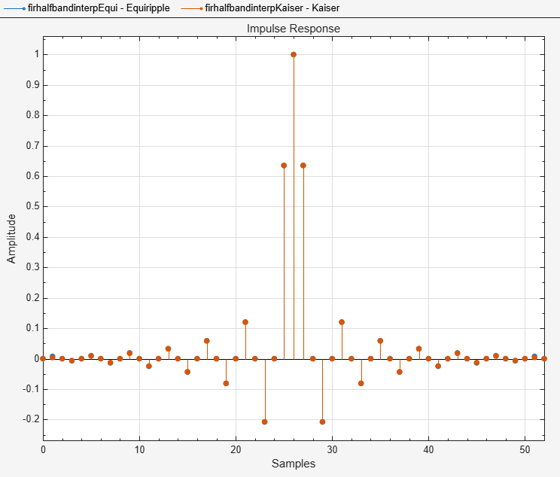Plot the magnitude and phase response.

If the filter specifications are tight, say a very high filter order with a very narrow transition width, the filter designed using the `"Kaiser"` method converges more effectively.

```hvftMag = fvtool(firhalfbandinterpEqui,firhalfbandinterpKaiser,... Analysis="Magnitude"); legend(hvftMag,{'Equiripple','Kaiser'})```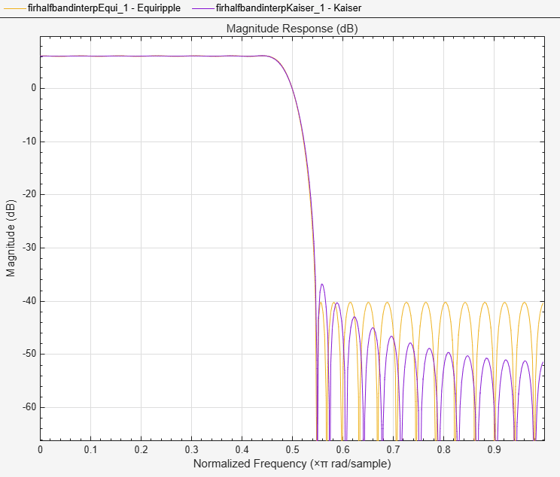```hvftPhase = fvtool(firhalfbandinterpEqui,firhalfbandinterpKaiser,... Analysis="Phase"); legend(hvftPhase,{'Equiripple','Kaiser'})```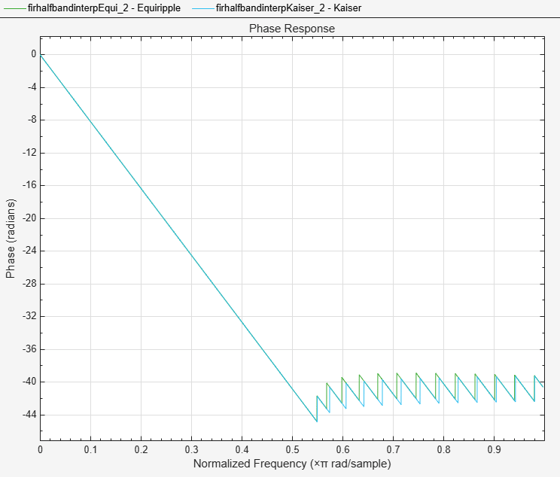Design an equiripple FIR halfband interpolator object of order 48 using the `designHalfbandFIR` function. Set the `Verbose` argument to `true`.

```hbFIR = designHalfbandFIR(FilterOrder=48,SystemObject=true,... Structure='interp',Verbose=true)```
```designHalfbandFIR(FilterOrder=48, TransitionWidth=0.1, DesignMethod="equiripple", Passband="lowpass", Structure="interp", SystemObject=true) ```
```hbFIR = dsp.FIRHalfbandInterpolator with properties: Specification: 'Coefficients' Numerator: [0 -0.0082 0 0.0079 0 -0.0116 0 0.0165 0 -0.0227 0 0.0309 0 -0.0419 0 0.0571 0 -0.0800 0 0.1193 0 -0.2073 0 0.6350 1 0.6350 0 -0.2073 0 0.1193 0 -0.0800 0 0.0571 0 -0.0419 0 0.0309 0 -0.0227 0 0.0165 0 -0.0116 ... ] (1x49 double) FilterBankInputPort: false Use get to show all properties ```

Create a `dsp.DynamicFilterVisualizer` object and visualize the magnitude response of the filter.

```dfv = dsp.DynamicFilterVisualizer(NormalizedFrequency=true); dfv(hbFIR);```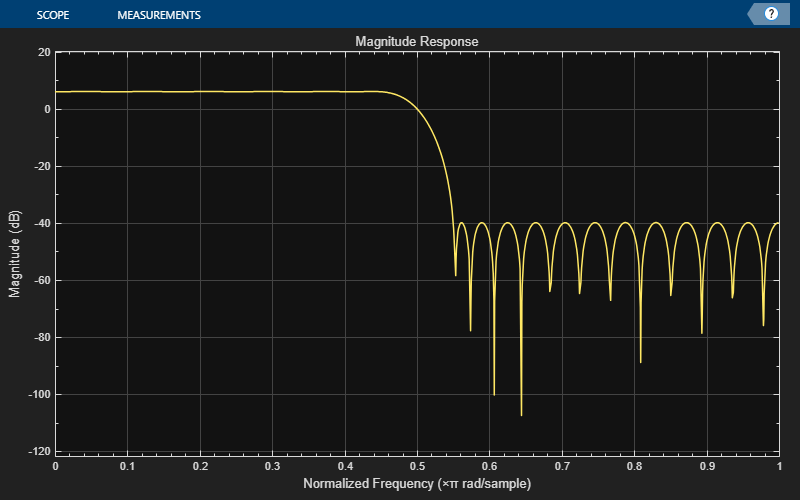The input is a cosine wave with an angular frequency of $\frac{\pi }{4}$ radians/sample.

`input = cos(pi/4*(0:39)');`

Interpolate the cosine signal using the FIR halfband interpolator.

`output = hbFIR(input);`

Plot the original and interpolated signals. In order to plot the two signals on the same plot, you must account for the output delay introduced by the FIR halfband interpolator and the scaling introduced by the filter. Use the `outputDelay` function to compute the `delay` value introduced by the interpolator. Shift the output by this delay value.

Visualize the input and the resampled signals. The input and output values coincide every other sample due to the interpolation factor of 2.

`[delay,FsOut] = outputDelay(hbFIR,FsIn=1)`
```delay = 12 ```
```FsOut = 2 ```
```nInput = (0:length(input)-1); tOutput = (0:length(output)-1)/FsOut-delay; stem(tOutput,output,'filled',MarkerSize=4); hold on; stem(nInput,input); hold off; xlim([-5,20]) legend('Interpolated by 2','Input signal','Location','best');```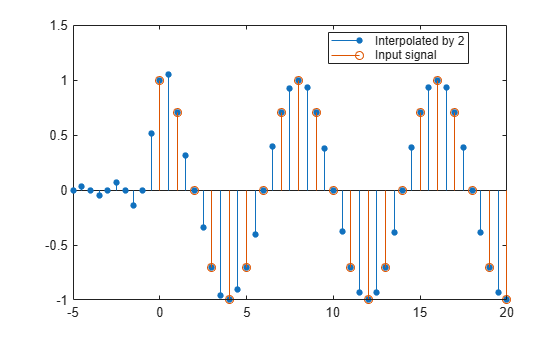Use a halfband analysis filter bank and interpolation filter to extract the low frequency subband from a speech signal.

Note: The `audioDeviceWriter` System object™ is not supported in MATLAB Online.

Set up the audio file reader, the analysis filter bank, audio device writer, and interpolation filter. The sample rate of the audio data is 22050 Hz. The order of the halfband filter is 52, with a transition width of 2 kHz.

Set the design method to `"Auto"`. This mode chooses one of the filter design methods, equiripple or Kaiser, based on the design parameters of the filter.

```afr = dsp.AudioFileReader(... "speech_dft.mp3",... SamplesPerFrame=1024); filtSpec = "Filter order and transition width"; Order = 52; TW = 2000; firhalfbanddecim = dsp.FIRHalfbandDecimator(... Specification=filtSpec,... FilterOrder=Order,... TransitionWidth=TW,... DesignMethod="Auto",... SampleRate=afr.SampleRate); firhalfbandinterp = dsp.FIRHalfbandInterpolator(... Specification=filtSpec,... FilterOrder=Order,... TransitionWidth=TW,... DesignMethod="Auto",... SampleRate=afr.SampleRate/2); adw = audioDeviceWriter(SampleRate=afr.SampleRate);```

View the magnitude response of the halfband filter.

`fvtool(firhalfbanddecim)`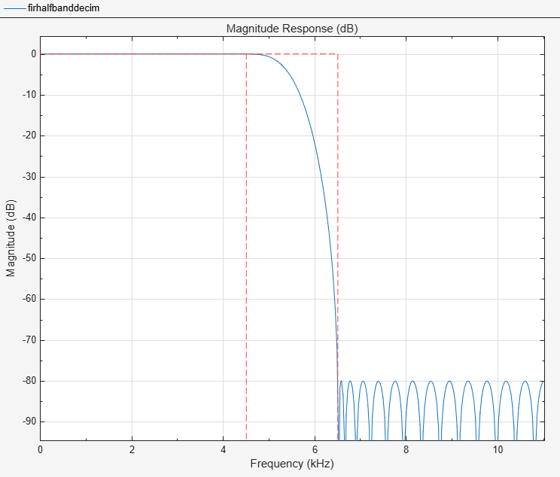Read the speech signal from the audio file in frames of 1024 samples. Filter the speech signal into lowpass and highpass subbands with a halfband frequency of 5512.5 Hz. Reconstruct a lowpass approximation of the speech signal by interpolating the lowpass subband. Play the filtered output.

```while ~isDone(afr) audioframe = afr(); xlo = firhalfbanddecim(audioframe); ylow = firhalfbandinterp(xlo); adw(ylow); end```

Wait until the audio file is played to the end, then close the input file and release the audio output resource.

```release(afr); release(adw);```

Use a halfband decimator and interpolator to implement a two-channel filter bank. This example uses an audio file input and shows that the power spectrum of the filter bank output does not differ significantly from the input.

Note: The `audioDeviceWriter` System object™ is not supported in MATLAB Online.

Set up the audio file reader and device writer. Construct the FIR halfband decimator and interpolator. Finally, set up the spectrum analyzer to display the power spectra of the filter-bank input and output.

```AF = dsp.AudioFileReader("speech_dft.mp3",SamplesPerFrame=1024); AP = audioDeviceWriter(SampleRate=AF.SampleRate); filterspec = "Filter order and transition width"; Order = 52; TW = 2000; firhalfbanddecim = dsp.FIRHalfbandDecimator(... Specification=filterspec,FilterOrder=Order,... TransitionWidth=TW,DesignMethod="Auto",... SampleRate=AF.SampleRate); firhalfbandinterp = dsp.FIRHalfbandInterpolator(... Specification=filterspec,FilterOrder=Order,... TransitionWidth=TW,SampleRate=AF.SampleRate/2,... DesignMethod="Auto",... FilterBankInputPort=true); SpecAna = spectrumAnalyzer(SampleRate=AF.SampleRate,... PlotAsTwoSidedSpectrum=false,... ShowLegend=true,... ChannelNames={'Input signal','Filtered output signal'});```

Read the audio 1024 samples at a time. Filter the input to obtain the lowpass and highpass subband signals decimated by a factor of two. This is the analysis filter bank. Use the halfband interpolator as the synthesis filter bank. Display the running power spectrum of the audio input and the output of the synthesis filter bank. Play the output.

```while ~isDone(AF) audioInput = AF(); [xlo,xhigh] = firhalfbanddecim(audioInput); audioOutput = firhalfbandinterp(xlo,xhigh); spectrumInput = [audioInput audioOutput]; SpecAna(spectrumInput); AP(audioOutput); end release(AF); release(AP); release(SpecAna);```Create a half-band interpolation filter. The filter order is 52 with a transition width of 0.0930 in normalized frequency units. Use the filter to upsample and interpolate a multichannel input.

Set the design method to `"Auto"`. This mode chooses one of the filter design methods, equiripple or Kaiser, based on the specified filter design parameters.

```Fs = 44.1e3; filterspec = "Filter order and transition width"; Order = 52; TW = 0.0930; firhalfbandinterp = dsp.FIRHalfbandInterpolator(... NormalizedFrequency=true,... Specification=filterspec,... FilterOrder=Order,... TransitionWidth=TW,... DesignMethod="Auto");```
```x = randn(1024,4); y = firhalfbandinterp(x);```